Mr Titmas

•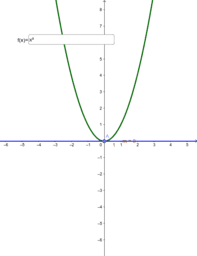Activity

Mr Titmas

•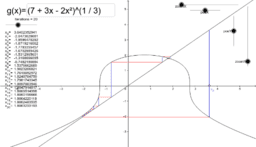Copy of Fixed Point Iteration: Rearrangement Method x=g(x)

Activity

Mr Titmas

•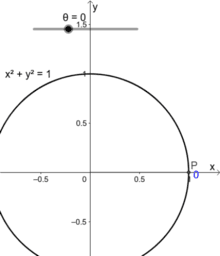Unit circle and trig graphs

Activity

Mr Titmas

•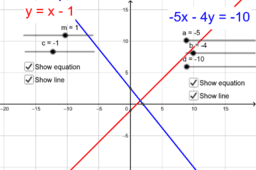Straight line graphs

Activity

Mr Titmas

•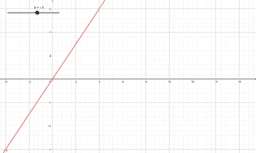Investigating direct proportion

Activity

Mr Titmas

•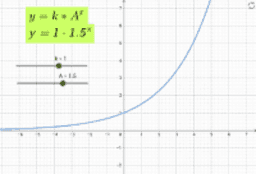Exponentials

Activity

Mr Titmas

•Activity

Mr Titmas

•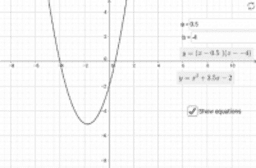Activity

Mr Titmas

•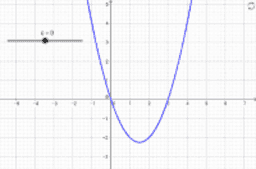Constant of integration example

Activity

Mr Titmas

•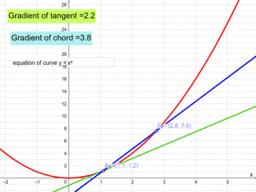Introducing differentiation

Activity

Mr Titmas

•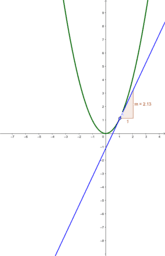Activity

Mr Titmas

•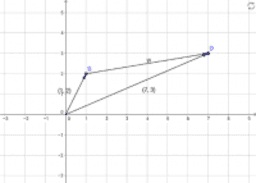Vecotr between two points

Activity

Mr Titmas

•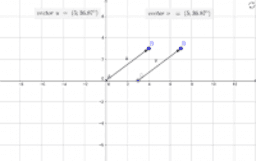Vector intro

Activity

Mr Titmas

•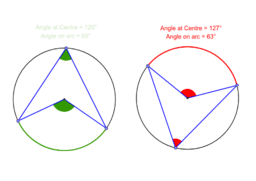Circle Theorems

Book

Mr Titmas

•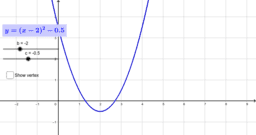Completing the square

Activity

Mr Titmas

•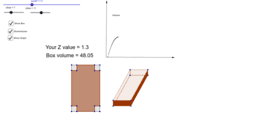Max box problem

Activity

Mr Titmas

•Parametric equations

Activity

Mr Titmas

•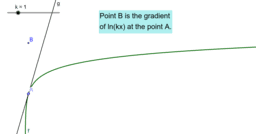Differentiating lnx

Activity

Mr Titmas

•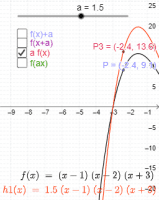Curve transformations

Activity

Mr Titmas

•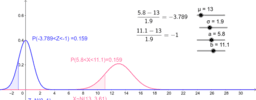Standardising Normal Distribution

Activity

Mr Titmas

•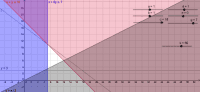Linear programming

Activity

Mr Titmas

•Drawing and recognising straight line graphs

Activity

Mr Titmas

•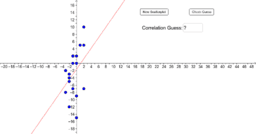Copy of Guess the Correlation!

Activity

Mr Titmas

•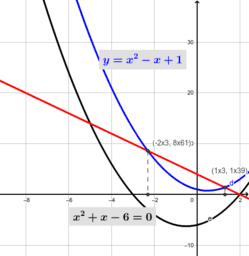Activity

Mr Titmas